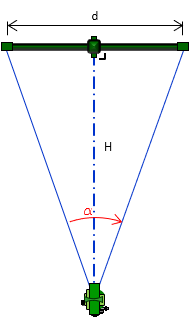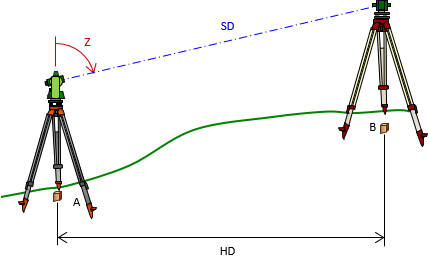## 1. Principle

### a. Tacheometry

Tacheometry is distance determination by passive optical methods. It uses an optical instrument at one end and an observable scale or known length at the other. It is passive because it is based on visual observation and geometry measurement and does not use artificial energy.

### b. Using a subtense bar

A subtense bar is one tacheometric method and the subject of this chapter. It is a physical bar with sighting targets at both ends. The distance, d,  between the targets is known, Figure C-1.Figure C-1Subtense Bar

The bar is set up on a tripod at one end of a line, centered on and perpendicular to it. At the other end is a transit or theodolite, Figure C-2.Figure C-2Set up

The horizontal angle between the two targets is measured with the transit/theodolite, Figure C-3.Figure C-3Measure Horizontal Angle

The slope distance, SD, between the transit./theodolite and subtense bar is computed using Equation C-1.Equation C-1

### c. Horizontal distance

The horizontal distance, HD, is obtained by measuring the zenith angle, Figure C-4, and using Equation C-2.Figure C-4Slope to HorizontalEquation C-2

### d. Vertical distance

Although primarily used for horizontal distance determination, a subtense bar can be used for establishing elevation, Figure C-5.Figure C-5Elevations by Subtense Bar

The vertical distance between the centers of the transit/theodolite and subtense bar can be computed using Equation C-3.Equation C-3

If the instrument is set over a point whose elevation is known and the heights of the instrument, HI, and subtense bar, HB, are measured, the ground elevation at the subtense bar can be computed from Equation C-4.Equation C-4

## 2. Application

Subtense bars pre-date electronic distance measurement and were typically used when direct taping between points was not possible. They were not universally adopted as a primary distance measuring device and many surveyors have never even seen a subtense bar. They are included here, not because anyone will use one today, but because they are an interesting way of distance determination.